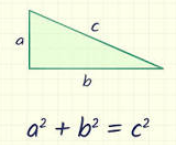# What is Pythagoras Theorem?

Pythagoras Theorem:

Pythagoras' theorem gives a fundamental relationship among the three sides of a right triangle. It states that the area of the square whose side is the hypotenuse is equal to the sum of the areas of the squares on the other two sides.

$a^2 + b^2 = c^2$; a, b are the legs and c the hypotenuse of the right triangle